# Using mouse and keyboard¶

In this section we look at using the mouse and keyboard to interact with shapes and bodies.

## Starting file¶

Our starting point is a file which alreaday has the:

• `App` class to create the application
• `Box` class to draw a static rectangular segment box
• `Circle` class to create dynamic circles

The `Box` class takes 2 diagonal points `p0` and `p1` and creates 4 static segments. The default is to place a box around the screen.

```class Box:
def __init__(self, p0=(0, 0), p1=(w, h), d=4):
x0, y0 = p0
x1, y1 = p1
ps = [(x0, y0), (x1, y0), (x1, y1), (x0, y1)]
for i in range(4):
segment = pymunk.Segment(b0, ps[i], ps[(i+1) % 4], d)
segment.elasticity = 1
segment.friction = 1
```

The program reacts to the

• `QUIT` button to close the window
• `Q` and `ESCAPE` key to end the application
• `P` key to save a screen capture under the name `mouse.png`
```    def do_event(self, event):
if event.type == QUIT:
self.running = False

elif event.type == KEYDOWN:
if event.key in (K_q, K_ESCAPE):
self.running = False

if event.key == K_p:
pygame.image.save(self.screen, 'mouse.png')
```

This code at the end of the file creates an empty box and runs the app:

```if __name__ == '__main__':
Box()
App().run()
```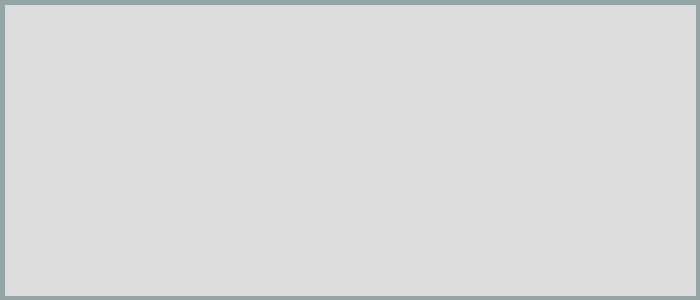`mouse0.py`

```import pymunk
from pymunk.pygame_util import *
from pymunk.vec2d import Vec2d

import pygame
from pygame.locals import *
import random

space = pymunk.Space()
b0 = space.static_body
size = w, h = 700, 300

GRAY = (220, 220, 220)
RED = (255, 0, 0)

class Circle:
self.body = pymunk.Body()
self.body.position = pos
shape.density = 0.01
shape.friction = 0.9
shape.elasticity = 1

class Box:
def __init__(self, p0=(0, 0), p1=(w, h), d=4):
x0, y0 = p0
x1, y1 = p1
ps = [(x0, y0), (x1, y0), (x1, y1), (x0, y1)]
for i in range(4):
segment = pymunk.Segment(b0, ps[i], ps[(i+1) % 4], d)
segment.elasticity = 1
segment.friction = 1

class App:
def __init__(self):
pygame.init()
self.screen = pygame.display.set_mode(size)
self.draw_options = DrawOptions(self.screen)
self.running = True

def run(self):
while self.running:
for event in pygame.event.get():
self.do_event(event)
self.draw()
space.step(0.01)

pygame.quit()

def do_event(self, event):
if event.type == QUIT:
self.running = False

elif event.type == KEYDOWN:
if event.key in (K_q, K_ESCAPE):
self.running = False

if event.key == K_p:
pygame.image.save(self.screen, 'mouse.png')

def draw(self):
self.screen.fill(GRAY)
space.debug_draw(self.draw_options)
pygame.display.update()

if __name__ == '__main__':
Box()
App().run()
```

## Create balls at random locations¶

We place 9 balls at random positions inside the box. In this example there is no gravity:

```if __name__ == '__main__':
Box()

r = 25
for i in range(9):
x = random.randint(r, w-r)
y = random.randint(r, h-r)
Circle((x, y), r)

App().run()
```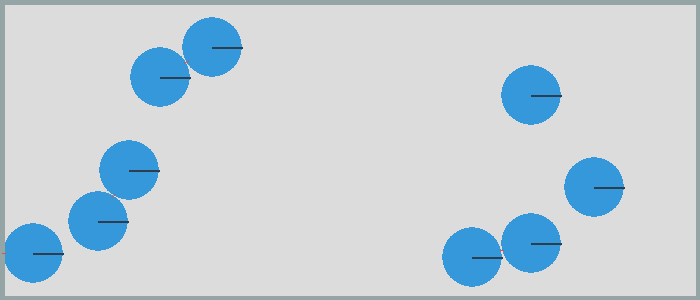## Select a ball with the mouse¶

Now let’s use a `MOUSEBUTTONDOWN` event to select an active shape with a mouse click. The two functions `from_pygame` and `to_pygame` allow us to change between

• `pygame` coordinates with the origin at the upper left
• `pymunk` coordinates with the origin at the lower left

The `point_query(p)` method checks if point `p` is inside the shape:

```elif event.type == MOUSEBUTTONDOWN:
p = from_pygame(event.pos, self.screen)
self.active_shape = None
for s in space.shapes:
dist, info = s.point_query(p)
if dist < 0:
self.active_shape = s
```

When there is an active shape, we surround it with a red circle:

```if self.active_shape != None:
s = self.active_shape
p = to_pygame(s.body.position, self.screen)
pygame.draw.circle(self.screen, RED, p, r, 3)
```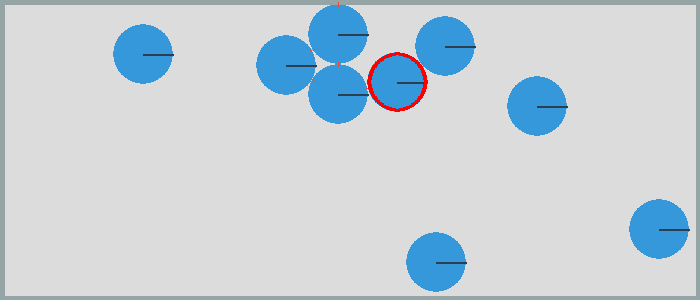## Move the active shape with keys¶

Let’s use the arrow keys to move the active object. For this we define a dictionary where we association the 4 direction unit vectors with the 4 arrow keys. If the key pressed is an arrow key, we move the active shape 20 pixels into that direction:

```keys = {K_LEFT: (-1, 0), K_RIGHT: (1, 0),
K_UP: (0, 1), K_DOWN: (0, -1)}
if event.key in keys:
v = Vec2d(keys[event.key]) * 20
if self.active_shape != None:
self.active_shape.body.position += v
```

## Rotate an object with the mouse¶

We can use the mouse-click into an object to change its angle. All we need to add is this line of code in the `MOUSEBUTTONDOWN` section:

```s.body.angle = (p - s.body.position).angle
```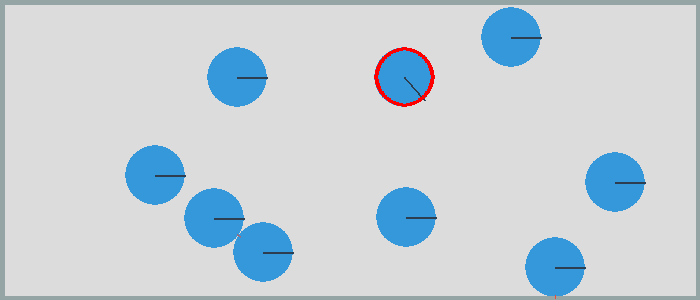## Pull a ball with the mouse¶

When releasing the mouse button, we take the mouse position and apply an impulse to the ball which is proportional to the red line drawn with the mouse, with `p0` being the object position and `p1` being the mouse position:

```elif event.type == MOUSEBUTTONUP:
if self.pulling:
self.pulling = False
b = self.active_shape.body
p0 = Vec2d(b.position)
p1 = from_pygame(event.pos, self.screen)
impulse = 100 * Vec2d(p0 - p1).rotated(-b.angle)
b.apply_impulse_at_local_point(impulse)
```

To draw the red line we add this to the drawing code:

```if self.active_shape != None:
b = self.active_shape.body
p0 = to_pygame(b.position, self.screen)
pygame.draw.circle(self.screen, RED, p0, r, 3)
if self.pulling:
pygame.draw.line(self.screen, RED, p0, self.p, 3)
pygame.draw.circle(self.screen, RED, self.p, r, 3)
```

Which results in this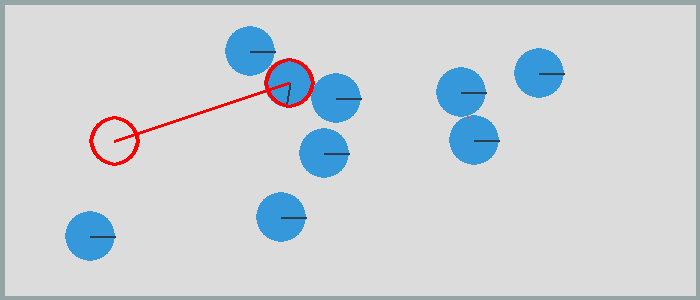## New objects at mouse position¶

Inside the `do_event()` section we add the following code:

```if event.key == K_c:
p = from_pygame(pygame.mouse.get_pos(), self.screen)
```

This will add smaller circles at the mouse position.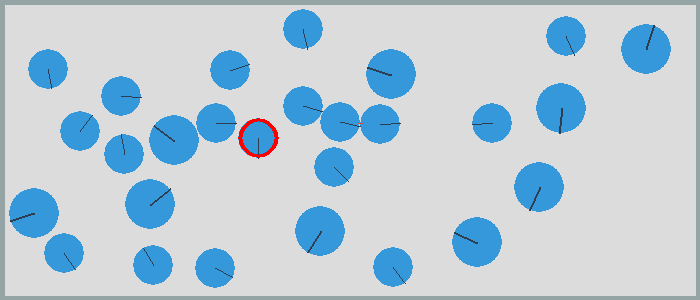## Remove an object¶

To remove the active object we add the following code:

```if event.key == K_BACKSPACE:
s = self.active_shape
if s != None:
space.remove(s, s.body)
self.active_shape = None
```

## Add a bounding box (BB)¶

Inside the `MOUSEBUTTONDOWN` section if clicking inside a shape, we add the following test to add the shape to the current selection if the cmd key is pressed:

```if pygame.key.get_mods() & KMOD_META:
self.selected_shapes.append(s)
print(self.selected_shapes)
else:
self.selected_shapes = []
```

In order to draw a shape’s bounding box (BB) we add the following method.

```    def draw_bb(self, shape):
pos = shape.bb.left, shape.bb.top
w = shape.bb.right - shape.bb.left
h = shape.bb.top - shape.bb.bottom
p = to_pygame(pos, self.screen)
pygame.draw.rect(self.screen, BLUE, (*p, w, h), 1)
```

In the App’s `draw()` section we add:

```for s in self.selected_shapes:
self.draw_bb(s)
```

This shows the currently selected objects with a bounding box.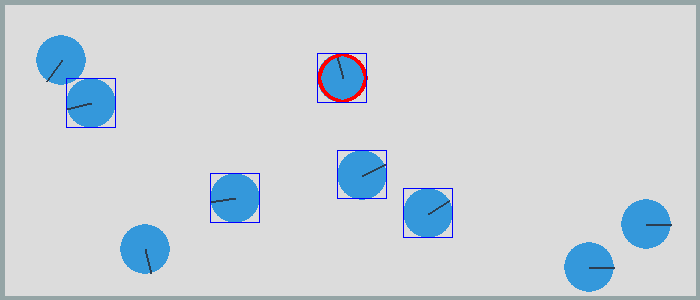## Toggle gravity¶

In order to turn on and off gravity we add the following code:

```elif event.key == K_g:
self.gravity = not self.gravity
if self.gravity:
space.gravity = 0, -900
else:
space.gravity = 0, 0
```

With gravity turned on, the circles fall to the ground.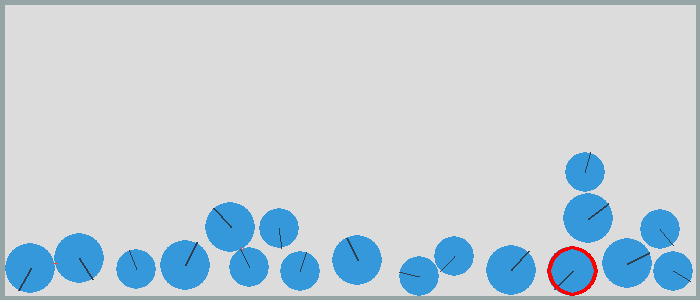## Animated GIF¶

Balls under the influence of gravity.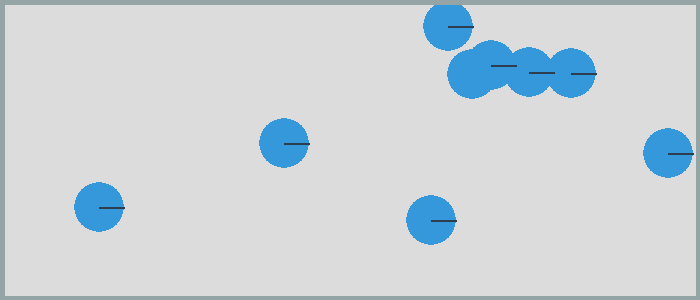Big and smalls balls.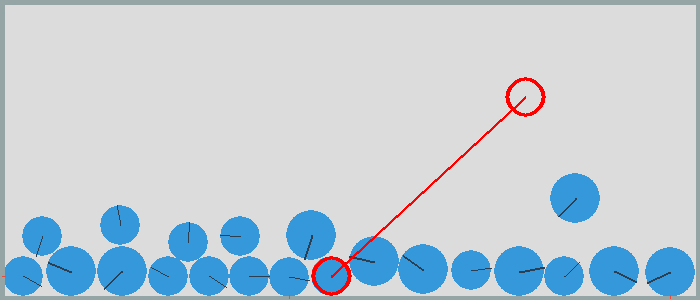## Complete source code¶

Here is the complete file.

`mouse.py`

```import pymunk
from pymunk.pygame_util import *
from pymunk.vec2d import Vec2d

import pygame
from pygame.locals import *

import math
import random
from PIL import Image

space = pymunk.Space()
b0 = space.static_body
size = w, h = 700, 300

GRAY = (220, 220, 220)
RED = (255, 0, 0)
BLUE = (0, 0, 255)

class Segment:
self.body = pymunk.Body()
self.body.position = p0
shape = pymunk.Segment(self.body, (0, 0), v, radius)
shape.density = 0.1
shape.elasticity = 0.5
shape.filter = pymunk.ShapeFilter(group=1)
shape.color = (0, 255, 0, 0)

class Circle:
self.body = pymunk.Body()
self.body.position = pos
shape.density = 0.01
shape.friction = 0.9
shape.elasticity = 1

class Box:
def __init__(self, p0=(0, 0), p1=(w, h), d=4):
x0, y0 = p0
x1, y1 = p1
ps = [(x0, y0), (x1, y0), (x1, y1), (x0, y1)]
for i in range(4):
segment = pymunk.Segment(b0, ps[i], ps[(i+1) % 4], d)
segment.elasticity = 1
segment.friction = 1

class App:
def __init__(self):
pygame.init()
self.screen = pygame.display.set_mode(size)
self.draw_options = DrawOptions(self.screen)
self.active_shape = None
self.selected_shapes = []
self.pulling = False
self.running = True
self.gravity = False
self.images = []
self.image_nbr = 60

def run(self):
while self.running:
for event in pygame.event.get():
self.do_event(event)
self.draw()
space.step(0.01)

pygame.quit()

def do_event(self, event):
if event.type == QUIT:
self.running = False

elif event.type == KEYDOWN:
if event.key in (K_q, K_ESCAPE):
self.running = False

elif event.key == K_p:
pygame.image.save(self.screen, 'mouse.png')

keys = {K_LEFT: (-1, 0), K_RIGHT: (1, 0),
K_UP: (0, 1), K_DOWN: (0, -1)}

if event.key in keys:
v = Vec2d(keys[event.key]) * 20
if self.active_shape != None:
self.active_shape.body.position += v

elif event.key == K_c:
p = from_pygame(pygame.mouse.get_pos(), self.screen)

elif event.key == K_BACKSPACE:
s = self.active_shape
if s != None:
space.remove(s, s.body)
self.active_shape = None

elif event.key == K_h:
self.gravity = not self.gravity
if self.gravity:
space.gravity = 0, -900
else:
space.gravity = 0, 0

elif event.key == K_g:
self.image_nbr = 60

elif event.type == MOUSEBUTTONDOWN:
p = from_pygame(event.pos, self.screen)
self.active_shape = None
for s in space.shapes:
dist, info = s.point_query(p)
if dist < 0:
self.active_shape = s
self.pulling = True

s.body.angle = (p - s.body.position).angle

if pygame.key.get_mods() & KMOD_META:
self.selected_shapes.append(s)
print(self.selected_shapes)
else:
self.selected_shapes = []

elif event.type == MOUSEMOTION:
self.p = event.pos

elif event.type == MOUSEBUTTONUP:
if self.pulling:
self.pulling = False
b = self.active_shape.body
p0 = Vec2d(b.position)
p1 = from_pygame(event.pos, self.screen)
impulse = 100 * Vec2d(p0 - p1).rotated(-b.angle)
b.apply_impulse_at_local_point(impulse)

def draw(self):
self.screen.fill(GRAY)
space.debug_draw(self.draw_options)

if self.active_shape != None:
s = self.active_shape
p = to_pygame(s.body.position, self.screen)
pygame.draw.circle(self.screen, RED, p, r, 3)
if self.pulling:
pygame.draw.line(self.screen, RED, p, self.p, 3)
pygame.draw.circle(self.screen, RED, self.p, r, 3)

for s in self.selected_shapes:
self.draw_bb(s)

if self.image_nbr > 0:
strFormat = 'RGBA'
raw_str = pygame.image.tostring(self.screen, strFormat, False)
image = Image.frombytes(strFormat, self.screen.get_size(), raw_str)
self.images.append(image)
self.image_nbr -= 1
if self.image_nbr == 0:
self.images.save('pillow.gif',
save_all=True, append_images=self.images[1:], optimize=False, duration=40, loop=0)
self.images = []

pygame.display.update()

def draw_bb(self, shape):
pos = shape.bb.left, shape.bb.top
w = shape.bb.right - shape.bb.left
h = shape.bb.top - shape.bb.bottom
p = to_pygame(pos, self.screen)
pygame.draw.rect(self.screen, BLUE, (*p, w, h), 1)

if __name__ == '__main__':
Box()

space.gravity = 0, -900
r = 25
for i in range(9):
x = random.randint(r, w-r)
y = random.randint(r, h-r)
Circle((x, y), r)

App().run()
```